Courses
Courses for Kids
Free study material
Free LIVE classes
More

# 2-Digit Subtraction for Class 1## What is Meant by 2-Digit Subtraction for Class 1?

Subtraction is one of the four basic arithmetic operations that we use not only in math but also in our day-to-day life. Subtraction is used when you go shopping; it is used when you give something to your sibling from your share, etc. Therefore, you can see that there are a lot of uses for this arithmetic operation in daily life. Subtraction can be of one digit, two digits, and so on. 1 digit subtraction is the case when two numbers with one digit are subtracted. 2-digit subtraction is the case where numbers with two digits are subtracted. Today we will learn 2-digit subtraction.

## What is a Two-digit Subtraction?

Have a look at the number below. The number is 14. This number is two-digit, and the digits are 1 and 4.

Therefore, a two-digit subtraction is a type of subtraction where either the numbers being subtracted are of two digits or the number being subtracted is of one digit, with the above number being of the two-digit type. We can use two methods for subtraction to solve 2-digit subtraction sums for class 1.

## The Types of Subtraction

We can perform 2-digit subtraction with the help of two methods, which are as follows:

### 1. Regrouping Method

Regrouping means you group the numbers into tens and ones. Therefore, the same thing is done for subtraction with regrouping.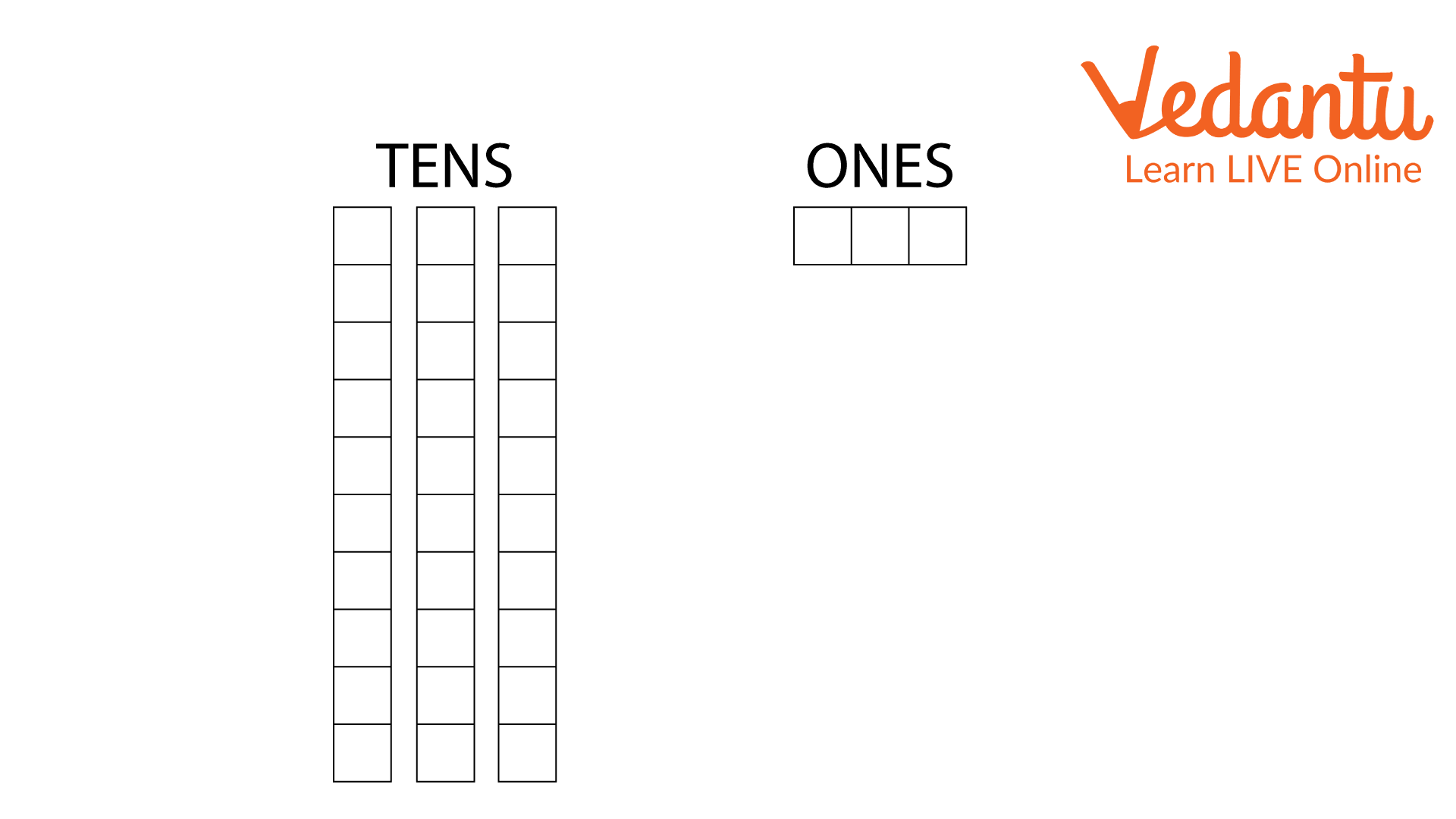33 Regrouped into Tens and Ones(self-made)

For example, subtract 17 from 33.

We will regroup 33 into tens and ones in the image above. You can see that under the tens group, there are three blocks with ten mini blocks. Under the ones, there are 3 mini blocks only. These mini blocks are the ones, and every block is 1 mini block.

When we perform subtraction, we subtract the ones from the ones and tens from the tens. You can see in the below image that since there are 3 ones and 7 ones to be subtracted, one block from the tens is brought to the one's column.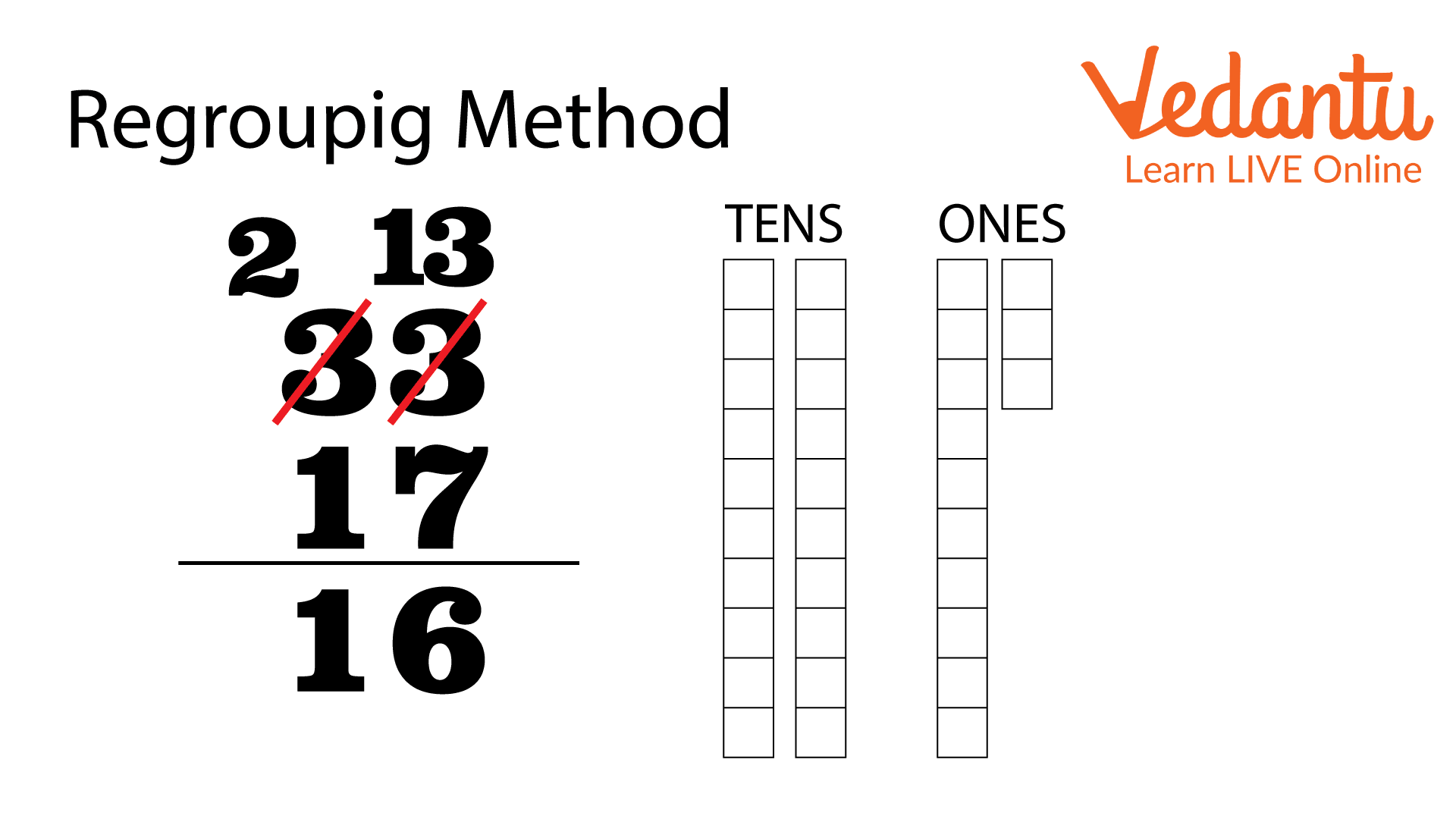Subtraction by Regrouping Method

There are 13 mini-blocks in one column, and we take away 7. The remaining number will be 6. Since one block from the tens has already been taken to the column, the remaining blocks in the tens are 2, and we have to subtract 1 from it. So, the result will be 1.

### 2. Without Regrouping Method

In the method without regrouping, one does not have to convert numbers into ones and tens. Instead, we can perform the subtraction directly. We write the number in the ones and tens of places.

For example,

Let us subtract 16 from 68. We have written the numbers in the ones, and tens place charts here.

Now, we can start from one’s place, i.e., from the right.

Here, we subtract 6 from 8.

The difference comes out to be 2 under the one’s column.

Then, we moved to the tent place.

Here, we subtract 1 from 6.

The difference is 5, and we write it under the tens column.

Hence, the difference is 52.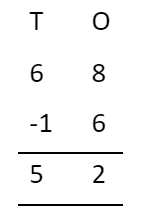This subtraction is performed without carrying from the tens place.

Therefore, this is not the carry method for subtraction for class 1.

Let us take another example.

Here, we will learn borrowing subtraction for class 1.

Let us subtract 15 from 53. We have written both numbers in the tens and ones chart.

Now, we can start from one’s place, i.e., from the right.

Here, we subtract 5 from 3. However, that is not possible.

Hence, we subtract by borrowing.

We borrow 1 from 5, which is in the tens’ place.

Hence, \$13 - 5 = 8\$

Now we move to tens.

Here, we subtract 1 from 4.

\$4 - 1 = 3\$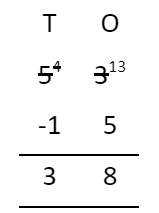The difference comes out to be 3, and we write it under the tens’ column.

The difference is 38, as you can see in the subtraction above.

## 2-Digit Subtraction Worksheet for Grade 1 with and without Borrowing

Here is a 2-digit number worksheet for subtraction.

Question-1 Subtract 11 from 34.

Solution:

Using the non-regrouping method, we first start from the right side and subtract 1 from 4.

\$4 - 1 = 3\$

The difference comes out to be 3.

We then proceed toward the numbers under the tens column.

We subtract 1 from 3.

\$3 - 1 = 2\$

The difference comes out to be 2.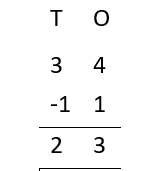Question-2 Subtraction of 15 from 42.

Solution:

Using the non-regrouping method, we first start from the right side and subtract 5 from 2 in the ones. However, we know that 5 is greater than 2. So, we borrow a 1 from 4 as 2-5 is impossible. So, we write, \$12 - 5 = 7\$

So, the difference is 7 in one’s place.

We then proceed toward the numbers under the tens column.

We subtract 1 from 3.

Because we already borrowed 1 from the tens column.

We write \$3 - 1 = 2\$

The difference is 2 in the tens column.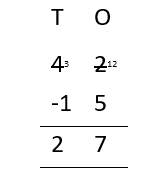Question-3 Subtraction of 20 from 45.

Solution:

Using the non-regrouping method, we first start from the right side and subtract 0 from 5.

\$5 - 0 = 5\$

The difference comes out to be 5.

We then proceed toward the numbers under the tens column.

We subtract 2 from 4.

\$4 - 2 = 2\$

The difference comes out to be 2.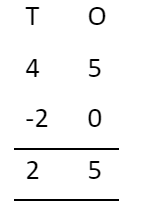## 2-Digit Subtraction Worksheet

Here is a free worksheet that you can use to practice more questions.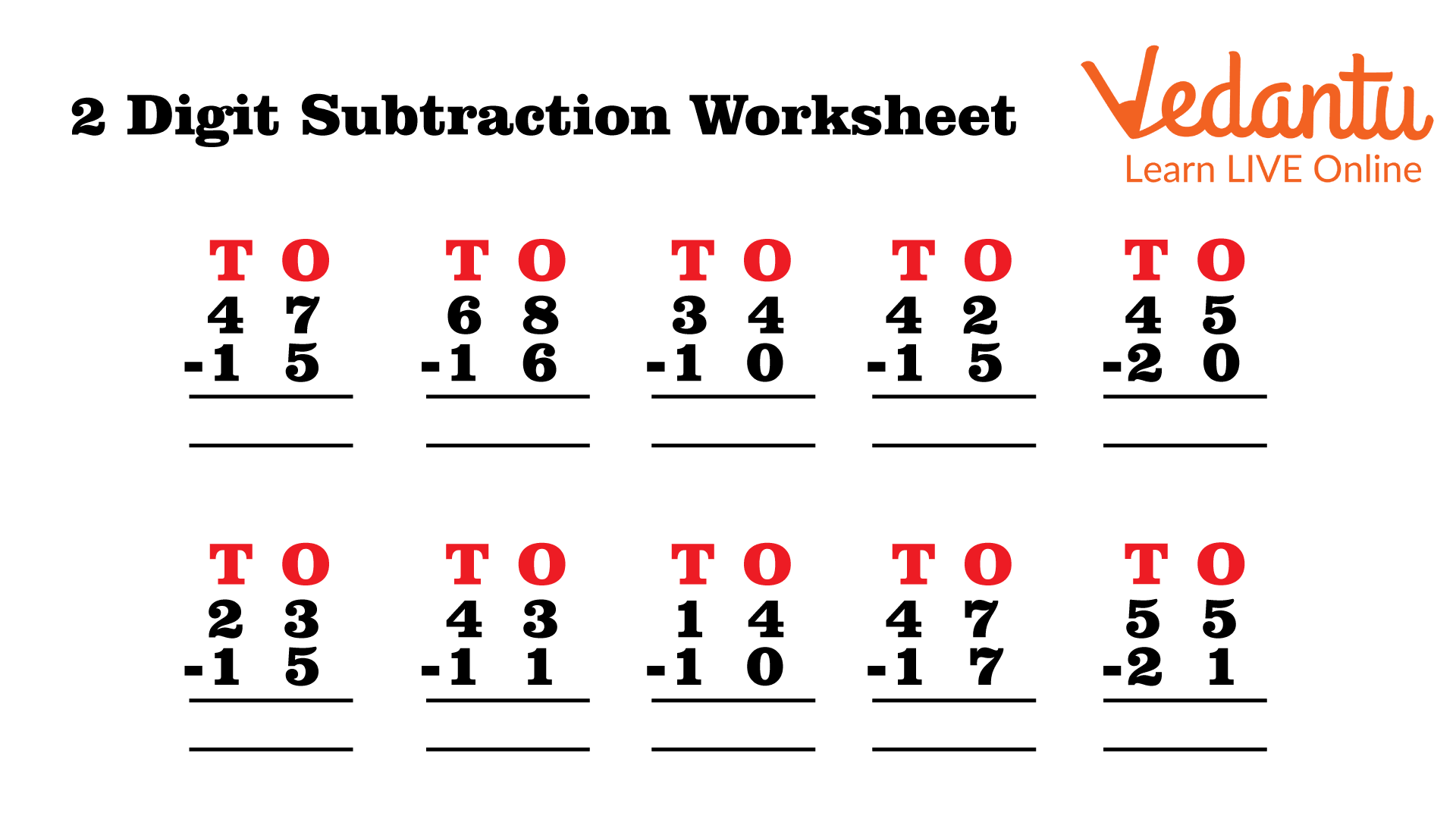2-digit Subtraction Worksheet for Class 1 (self-made)

## Conclusion

You have learned about subtraction in this article, specifically two-digit subtraction. Apart from these, you have also learned about the two methods for solving 2-digit subtraction; subtraction with and without regrouping, and some solved questions. Next time, you will learn more about other arithmetic operations.

Last updated date: 26th Sep 2023
Total views: 94.2k
Views today: 2.94k

## FAQs on 2-Digit Subtraction for Class 1

1. How can we use the 2-digit subtraction worksheets?

2-digit subtraction worksheets for kids can be used by either copying the questions in the worksheets or downloading them and directly practicing them. Worksheets are one of the best ways to both learn the concepts and help practice. After understanding the solved examples, kids can easily practice the questions given in the worksheet.

2. How to make subtraction fun?

We can use card games and real-life examples of subtraction, like food items, to attract children to learn subtraction. For example, suppose you give the child 10 candies and ask them to give 5 candies to their sibling. In this way, you can help the child understand the meaning of subtraction, thereby introducing the concept to the child.

3. Where is the regrouping method more useful?

The regrouping method is proper when the minuend is smaller than the subtrahend. For example, you bought something for 25 INR and gave 30 INR to the shopkeeper. In that case, regrouping is of great help. In that case, regrouping is of great help. Since 0 is less than 5, you must use the regrouping method to take 1 ten and convert it to ones.